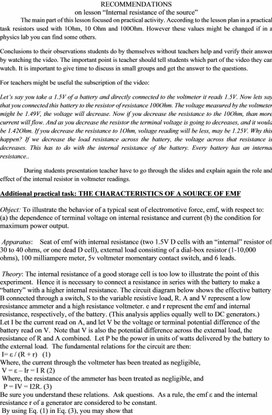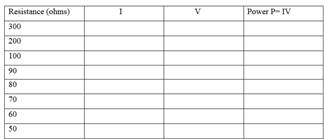PHY_10_40_V1_TG_Internal r
Оценка 4.7

# PHY_10_40_V1_TG_Internal r

Оценка 4.7
docx
07.05.2020PHY_10_40_V1_TG_Internal r.docx

RECOMMENDATIONS

on lesson “Internal resistance of the source”

The main part of this lesson focused on practical activity. According to the lesson plan in a practical task resistors used with 1Ohm, 10 Ohm and 100Ohm. However these values might be changed if in a physics lab you can find some others.

Conclusions to their observations students do by themselves without teachers help and verify their answer by watching the video. The important point is teacher should tell students which part of the video they can watch. It is important to give time to discuss in small groups and get the answer to the questions.

For teachers might be useful the subscription of the video:

Let’s say you take a 1.5V of a battery and directly connected to the voltmeter it reads 1.5V. Now lets say that you connected this battery to the resistor of resistance 100Ohm. The voltage measured by the voltmeter might be 1.49V, the voltage will decrease. Now if you decrease the resistance to the 10Ohm, than more current will flow. And as you decrease the resistor the terminal voltage is going to decreases, and it would be 1.42Ohm. If you decrease the resistance to 1Ohm, voltage reading will be less, may be 1.25V. Why this happen? If we decrease the load resistance across the battery, the voltage across that resistance is decreases. This has to do with the internal resistance of the battery. Every battery has an internal resistance..

During students presentation teacher have to go through the slides and explain again the role and effect of the internal resistor in voltmeter readings.

Object: To illustrate the behavior of a typical seat of electromotive force, emf, with respect to:  (a) the dependence of terminal voltage on internal resistance and current (b) the condition for maximum power output.

Apparatus:    Seat of emf with internal resistance (two 1.5V D cells with an “internal” resistor of 30 to 40 ohms, or one dead D cell), external load consisting of a dial-box resistor (1-10,000 ohms), 100 milliampere meter, 5v voltmeter momentary contact switch, and 6 leads.

Theory: The internal resistance of a good storage cell is too low to illustrate the point of this experiment.  Hence it is necessary to connect a resistance in series with the battery to make a “battery” with a higher internal resistance. The circuit diagram below shows the effective battery B connected through a switch, S to the variable resistive load, R. A and V represent a low resistance ammeter and a high resistance voltmeter. e and r represent the emf and internal resistance, respectively, of the battery. (This analysis applies equally well to DC generators.)

Let I be the current read on A, and let V be the voltage or terminal potential difference of the battery read on V.  Note that V is also the potential difference across the external load, the resistance of R and A combined.  Let P be the power in units of watts delivered by the battery to the external load.  The fundamental relations for the circuit are then:

I= ε / (R + r)   (1)

Where, the current through the voltmeter has been treated as negligible,

V = ε – Ir = I R (2)

Where, the resistance of the ammeter has been treated as negligible, and

P = IV = I2R. (3)

Be sure you understand these relations.  Ask questions.  As a rule, the emf ε and the internal resistance r of a generator are considered to be constant.

By using Eq. (1) in Eq. (3), you may show that

P = ε2 R / (R +r)2. (4)

Note that P approaches zero both for very small and for very large values of load resistance R.  Hence the power delivered will be a maximum for some intermediate load resistance.  To find the value of R which results in maximum power, one differentiates Eq. (4) with respect to R and equates the derivative to zero.  The solution of this relation yields

R = r, for P at maximum. (5)

Under these conditions, the load is said to be “matched” to the seat of emf.

Although P is now a maximum, the power (I2r) dissipated in the battery or generator is as great as the power (I2R) delivered to the load.  Hence this arrangement is not ordinarily used with batteries or generators.  This principle is however of fundamental importance in many circuit applications, as in “matching” speakers to audio amplifiers.

Procedure:  Connect the circuit components, except for one battery lead; see that all contacts are firm.  After the circuit has been checked by the instructor, set R to about 300 ohms and close S.  Tabulate the readings of A and V and P for a series of decreasing values of R including a column for P.  By computing P as you proceed, the changes in R may be made in suitable steps.  These should be large at first (100 or 200 ohms) but much smaller (5or 10 ohms) as R approaches r in value.  You should change R only 1 or 2 ohms at a step in the vicinity of Pmax.

Report:  Plot the following graphs, each on a full sheet.

1) V as a function of I.

2) P as a function of R for values of R up to 100 ohms.

Data:Questions:

1) Show that Eq. (4) follows from Eq. (1) and (3), and that Eq. (4) with Eq. (5) gives Pmax = ε 2/4R.

2) Determine the experimental values for E and r using the data from the first graph and equation (2).  From the second graph, read Pmax and compare with ε 2/4r.  Read R for Pmax and compare with r.

3) Show that at maximum power output, the terminal voltage of the battery is half its emf.

4) Why is a dead battery characterized by a high internal resistance?

5) Explain why batteries of low current capacity have higher internal resistances than those batteries of high current capacity, e.g., a 12 v flashlight battery vs. a 12 v car battery.

Useful resources: